### Home > CALC > Chapter 4 > Lesson 4.1.1 > Problem4-8

4-8.
1. For each function, find its slope function, f ′(x). Homework Help ✎

1.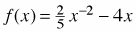2.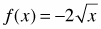3. f(x) = 6 cos x

4.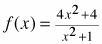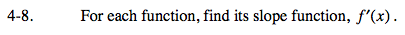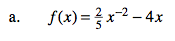Power Rule.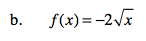Before you differentiate, rewrite f(x) with a fractional exponent.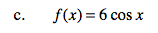Will f '(x) be positive or negative?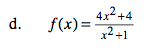Before you differentiate, factor the numerator and simplify. THINK: Will the derivative need a restricted domain? Why or why not?